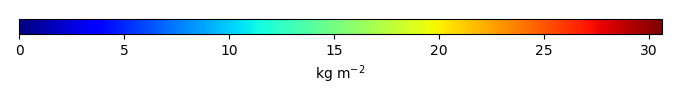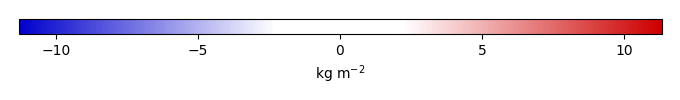# Mean State

Period Mean (original grids) [Pg]
Model Period Mean (intersection) [Pg]
Model Period Mean (complement) [Pg]
Benchmark Period Mean (intersection) [Pg]
Benchmark Period Mean (complement) [Pg]
Bias [kg m-2]
Bias Score 
Spatial Distribution Score 
Overall Score 
Benchmark [-] 38.7
CLM4 [-] 49.5 50.3 0.175 38.7 0.0457 2.84 0.647 0.812 0.729
CLM4.5 [-] 45.4 45.4 0.214 38.7 0.0457 1.81 0.606 0.776 0.691
CLM5 [-] 38.5 38.7 0.202 38.7 0.0457 0.293 0.774 0.952 0.863
Period Mean (original grids) [Pg]
Model Period Mean (intersection) [Pg]
Model Period Mean (complement) [Pg]
Benchmark Period Mean (intersection) [Pg]
Benchmark Period Mean (complement) [Pg]
Bias [kg m-2]
Bias Score 
Spatial Distribution Score 
Overall Score 
Benchmark [-] 11.9
CLM4 [-] 7.62 6.82 0.00883 11.9 0.0196 -0.754 0.580 0.883 0.731
CLM4.5 [-] 2.26 2.02 0.0241 11.9 0.0196 -1.47 0.428 0.379 0.404
CLM5 [-] 3.25 2.85 0.0430 11.9 0.0196 -1.35 0.454 0.594 0.524
Period Mean (original grids) [Pg]
Model Period Mean (intersection) [Pg]
Model Period Mean (complement) [Pg]
Benchmark Period Mean (intersection) [Pg]
Benchmark Period Mean (complement) [Pg]
Bias [kg m-2]
Bias Score 
Spatial Distribution Score 
Overall Score 
Benchmark [-] 24.6
CLM4 [-] 10.9 10.4 0.239 24.5 0.0612 -1.42 0.526 0.842 0.684
CLM4.5 [-] 4.94 4.60 0.227 24.5 0.0612 -2.04 0.441 0.697 0.569
CLM5 [-] 12.3 11.7 0.367 24.5 0.0612 -1.21 0.507 0.799 0.653
Period Mean (original grids) [Pg]
Model Period Mean (intersection) [Pg]
Model Period Mean (complement) [Pg]
Benchmark Period Mean (intersection) [Pg]
Benchmark Period Mean (complement) [Pg]
Bias [kg m-2]
Bias Score 
Spatial Distribution Score 
Overall Score 
Benchmark [-] 36.8
CLM4 [-] 62.7 60.1 2.37 35.7 1.10 12.8 0.454 0.682 0.568
CLM4.5 [-] 54.1 51.6 2.16 35.7 1.10 10.3 0.535 0.872 0.703
CLM5 [-] 39.9 37.9 1.80 35.7 1.10 5.14 0.715 0.918 0.817
Period Mean (original grids) [Pg]
Model Period Mean (intersection) [Pg]
Model Period Mean (complement) [Pg]
Benchmark Period Mean (intersection) [Pg]
Benchmark Period Mean (complement) [Pg]
Bias [kg m-2]
Bias Score 
Spatial Distribution Score 
Overall Score 
Benchmark [-] 38.4
CLM4 [-] 40.6 30.7 10.5 38.3 0.0891 -0.539 0.580 0.644 0.612
CLM4.5 [-] 40.9 30.9 10.3 38.3 0.0891 -0.477 0.530 0.603 0.567
CLM5 [-] 31.6 23.2 9.15 38.3 0.0891 -1.53 0.586 0.801 0.694
Period Mean (original grids) [Pg]
Model Period Mean (intersection) [Pg]
Model Period Mean (complement) [Pg]
Benchmark Period Mean (intersection) [Pg]
Benchmark Period Mean (complement) [Pg]
Bias [kg m-2]
Bias Score 
Spatial Distribution Score 
Overall Score 
Benchmark [-] 43.7
CLM4 [-] 28.1 26.8 0.491 43.4 0.257 -1.57 0.607 0.856 0.731
CLM4.5 [-] 22.9 21.6 0.572 43.4 0.257 -2.15 0.527 0.785 0.656
CLM5 [-] 24.8 23.2 0.670 43.4 0.257 -1.98 0.606 0.890 0.748
Period Mean (original grids) [Pg]
Model Period Mean (intersection) [Pg]
Model Period Mean (complement) [Pg]
Benchmark Period Mean (intersection) [Pg]
Benchmark Period Mean (complement) [Pg]
Bias [kg m-2]
Bias Score 
Spatial Distribution Score 
Overall Score 
Benchmark [-] 13.7
CLM4 [-] 7.61 1.66 5.85 13.5 0.139 -1.85 0.418 0.581 0.499
CLM4.5 [-] 7.63 1.55 6.01 13.5 0.139 -1.85 0.413 0.544 0.479
CLM5 [-] 11.0 3.66 7.16 13.5 0.139 -1.38 0.445 0.638 0.541
Period Mean (original grids) [Pg]
Model Period Mean (intersection) [Pg]
Model Period Mean (complement) [Pg]
Benchmark Period Mean (intersection) [Pg]
Benchmark Period Mean (complement) [Pg]
Bias [kg m-2]
Bias Score 
Spatial Distribution Score 
Overall Score 
Benchmark [-] 352.
CLM4 [-] 467. 376. 90.9 348. 3.81 0.986 0.539 0.628 0.583
CLM4.5 [-] 452. 327. 125. 348. 3.81 0.359 0.537 0.704 0.621
CLM5 [-] 486. 309. 178. 348. 3.81 0.0653 0.638 0.854 0.746
Period Mean (original grids) [Pg]
Model Period Mean (intersection) [Pg]
Model Period Mean (complement) [Pg]
Benchmark Period Mean (intersection) [Pg]
Benchmark Period Mean (complement) [Pg]
Bias [kg m-2]
Bias Score 
Spatial Distribution Score 
Overall Score 
Benchmark [-] 2.53
CLM4 [-] 1.32 0.117 1.21 2.53 0.00250 -1.09 0.385 0.0111 0.198
CLM4.5 [-] 1.34 0.119 1.24 2.53 0.00250 -1.08 0.385 0.0203 0.203
CLM5 [-] 1.55 0.211 1.35 2.53 0.00250 -1.04 0.395 0.0211 0.208
Period Mean (original grids) [Pg]
Model Period Mean (intersection) [Pg]
Model Period Mean (complement) [Pg]
Benchmark Period Mean (intersection) [Pg]
Benchmark Period Mean (complement) [Pg]
Bias [kg m-2]
Bias Score 
Spatial Distribution Score 
Overall Score 
Benchmark [-] 11.1
CLM4 [-] 4.64 4.09 0.284 10.9 0.149 -1.52 0.455 0.665 0.560
CLM4.5 [-] 8.47 7.78 0.434 10.9 0.149 0.0277 0.535 0.684 0.610
CLM5 [-] 10.2 9.38 0.547 10.9 0.149 0.813 0.607 0.775 0.691
Period Mean (original grids) [Pg]
Model Period Mean (intersection) [Pg]
Model Period Mean (complement) [Pg]
Benchmark Period Mean (intersection) [Pg]
Benchmark Period Mean (complement) [Pg]
Bias [kg m-2]
Bias Score 
Spatial Distribution Score 
Overall Score 
Benchmark [-] 1.55
CLM4 [-] 13.4 2.27 11.0 1.52 0.0247 1.54 0.638 0.767 0.703
CLM4.5 [-] 14.7 1.63 12.9 1.52 0.0247 0.864 0.600 0.764 0.682
CLM5 [-] 11.9 1.32 10.5 1.52 0.0247 0.434 0.550 0.683 0.617
Period Mean (original grids) [Pg]
Model Period Mean (intersection) [Pg]
Model Period Mean (complement) [Pg]
Benchmark Period Mean (intersection) [Pg]
Benchmark Period Mean (complement) [Pg]
Bias [kg m-2]
Bias Score 
Spatial Distribution Score 
Overall Score 
Benchmark [-] 0.219
CLM4 [-] 3.99 0.100 3.95 0.219 -0.388 0.491 0.192 0.341
CLM4.5 [-] 2.99 0.0814 2.95 0.219 -0.445 0.467 0.128 0.297
CLM5 [-] 3.04 0.0844 2.99 0.219 -0.433 0.468 0.0819 0.275
Period Mean (original grids) [Pg]
Model Period Mean (intersection) [Pg]
Model Period Mean (complement) [Pg]
Benchmark Period Mean (intersection) [Pg]
Benchmark Period Mean (complement) [Pg]
Bias [kg m-2]
Bias Score 
Spatial Distribution Score 
Overall Score 
Benchmark [-] 100.
CLM4 [-] 159. 158. 0.331 100. 0.0690 7.20 0.511 0.493 0.502
CLM4.5 [-] 141. 141. 0.404 100. 0.0690 5.17 0.585 0.674 0.630
CLM5 [-] 128. 128. 0.396 100. 0.0690 3.63 0.709 0.919 0.814
Period Mean (original grids) [Pg]
Model Period Mean (intersection) [Pg]
Model Period Mean (complement) [Pg]
Benchmark Period Mean (intersection) [Pg]
Benchmark Period Mean (complement) [Pg]
Bias [kg m-2]
Bias Score 
Spatial Distribution Score 
Overall Score 
Benchmark [-] 18.4
CLM4 [-] 17.8 17.9 0.122 18.4 0.0281 0.0899 0.547 0.530 0.539
CLM4.5 [-] 13.5 13.4 0.195 18.4 0.0281 -0.573 0.515 0.570 0.543
CLM5 [-] 21.8 21.8 0.347 18.4 0.0281 0.735 0.546 0.579 0.563
Period Mean (original grids) [Pg]
Model Period Mean (intersection) [Pg]
Model Period Mean (complement) [Pg]
Benchmark Period Mean (intersection) [Pg]
Benchmark Period Mean (complement) [Pg]
Bias [kg m-2]
Bias Score 
Spatial Distribution Score 
Overall Score 
Benchmark [-] 7.28
CLM4 [-] 4.80 5.04 0.0866 7.15 0.128 -0.164 0.492 0.496 0.494
CLM4.5 [-] 5.00 5.06 0.113 7.15 0.128 -0.0225 0.468 0.509 0.488
CLM5 [-] 6.25 6.14 0.145 7.15 0.128 0.387 0.524 0.568 0.546

# Temporally integrated period mean

BENCHMARK MEANMODEL MEANBIASBIAS SCORESPATIAL TAYLOR DIAGRAMMODEL COLORS# Spatially integrated regional mean

MODEL COLORS# All Models

BenchmarkCLM4CLM4.5CLM5# Data Information

title: Global Tropical Forest Biomass

institution: NASA-JPL (NASA Jet Propulsion Laboratory, California Institute of Technology, Pasadena, California 91109, USA)

institute_id: NASA-JPL

source: 4079 in situ inventory plots, satellite light detection and ranging (LiDAR) samples of forest structure, and optical and microwave imagery (1-km resolution) to extrapolate estimates

history: 2015-01-08-T06:37:41Z Converted from 1 km x 1 km to 0.5 degree x 0.5 degree by Mingquan Mu (mmu@uci.edu) on Fri Jan 9 10:37:41 PST 2015

creation_date: 2015-01-08-T06:37:41Z

Conventions: CF-1.6

product: observations

data_structure: grid

realm: land

contact: Prof. James T. Randerson (jranders@uci.edu) or Dr. Mingquan Mu (mmu@uci.edu)

references: Saatchi, S. S., N. L. Harris, S. Brown, M. Lefsky, E. T. A. Mitchard, W. Salas, B. R. Zutta, W. Buermann, S. L. Lewis, S. Hagen, S. Petrova, L. White, M. Silman, and A. Morel (2011), Benchmark map of forest carbon stocks in tropical regions across three continents, Proc. Nat. Acad. Sci., 108(24):9899-9904, doi:10.1073/pnas.1019576108.